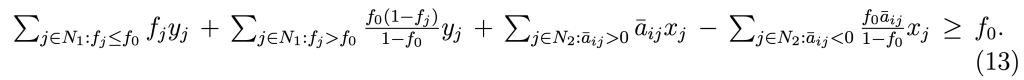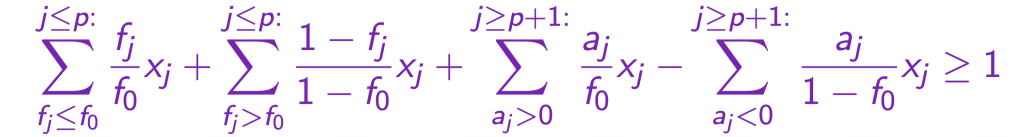With ojAlgo v51.2.0 the `IntegerSolver` gained support for Gomory Mixed Integer (GMI) cuts.

Details of what they are and how they’re derived is described in many publications. Just google it. When/if you do; I recommend reading stuff published by John E. Mitchell. In particular The Gomory Mixed Integer Cut, by John E. Mitchell is a good description of the derivation of the Gomory mixed integer cuts.

Gomory (Mixed Integer) cuts are named after Ralph E. Gomory. He came up with an algorithm to solve integer linear programs (ILP) – Gomory’s Cutting Plane Algorithm. It iteratively solved a series of LP:s generating cuts (cutting planes) in-between each iteration. The cuts “remove” more and more of the non-integer solution space so that the algorithm eventually ends up with an integer solution. It only worked for pure integer programs (all variables integer) and didn’t have very good numerical properties. Later he came up with a modified version that handled a mix of integer and non-integer variables. This required a different formula to generate the cuts. Funny thing is that the mixed integer cuts are equal to or “better” than the pure integer cuts even when applied to pure integer models. So, in practice, it is always better to derive the cuts using the mixed integer formula, even if the model is a pure integer model. Strangely most published examples describe the original pure integer algorithm and cuts. Sure, it came first and is slightly easier to derive, but the mixed integer variant is not much more difficult and it is what’s used in practice.

Apart from announcing the fact that the ojAlgo `IntegerSolver` now makes use of GMI cuts. this post aims to demonstrate the generation of such cuts.

This is the GMI cut formula as it is often derived:Copied from a pdf (probably lecture notes) titled The Gomory Mixed Integer Cut, by John E. Mitchell

This variation is, I believe, how it is mostly used:Copied from a presentation slides titled Lecture 2, Split Inequalities and Gomory Mixed Integer Cuts, by Gérard Cornuéjols

There are 4 sets of coefficients – easily identified in both the formulas above, even if the two authors use different indices/set declarations. Primarily the integer and non-integer variables are differentiated. The non-integer variables are further separated depending on if their coefficients in the equation are positive or negative. Among the integer variables one is special, and the others are separated depending on how they relate to that special one. This is where you should get the details from some other publication. I recommend The Gomory Mixed Integer Cut, by John E. Mitchell.

To generate a GMI cut one starts with a valid equality in nonnegative integer variables and nonnegative continuous variables. The simplex tableau obtained from solving the relaxed LP is a perfect place to find such equalities. Just pick any row corresponding to a basic integer variable at a non-integral value.

Since a simplex tableau typically contains a multitude of slack variables it is important to keep track of which of those are also restricted to be integer – that’s important to know when applying the formula to generate the cuts.

Gomory’s Cutting Plane Algorithm didn’t work very well. Due to numerical issues it often did not converge to a solution. But, many years later, the building blocks of the algorithm – the cuts – were incorporated in branch-and-bound algorithms typically used to solve MIP models. Instead of trying to solve the entire problem using only cuts, the cuts where selectively used to reduce the size of the branch-and-bound tree. This resulted in huge speed-ups of those algorithms.

ojAlgo’s `IntegerSolver` generates a set of cuts at the root of the branch-and-bound tree, and then additional cuts at intervals when certain conditions are met.

To test the GMI cut generation (primarily during initial development) we also created a `GomorySolver` – implementing Gomory’s Mixed Integer Cutting Plane Algorithm. It works for some small/simple cases. Below is example code using that solver to solve a problem taken from Branch-and-Cut Algorithms for Combinatorial Optimization Problems by John E. Mitchell.

## Console Output

```class GomoryMixedIntegerCuts
ojAlgo
2022-04-20

The model to solve
############################################
0 <= x1 (-6)
0 <= x2 (-5)
EXPR1: 0.0 <= 5
EXPR0: 0.0 <= 11
############################################

Solve with progress output

Iteration 1: { 2.428571428571429, 3.7142857142857144 }

Iteration 2: { 2.3333333333619044, 3.0 }

Iteration 3: { 2.9999999999833333, 2.00000000005 }

OPTIMAL -28.0 @ { 3, 2 }

Solve again with more detailed debug output

Iteration 1: { 2.428571428571429, 3.7142857142857144 }

2.428571428571429 ! Integer: x1
CUT_GMI_1_4: -4.2 < [1=-1.39999999999]
CUT_GMI_0_5: -7.58333333334 < [0=-1.74999999999, 1=-1.16666666666]
Iteration 2: { 2.3333333333619044, 3.0 }

2.3333333333619044 ! Integer: x1
CUT_GMI_0_6: -14.9999999988 < [0=-2.99999999974, 1=-2.99999999974]
Iteration 3: { 2.9999999999833333, 2.00000000005 }

OPTIMAL -28.0 @ { 3, 2 }

############################################
0 <= x1: 3 (-6) <= 3
0 <= x2: 2 (-5) <= 4
EXPR1: 1.0 <= 5
EXPR0: 11.0 <= 11
############################################
```

## A Closer Look At Cut Generation

After the 2:d iteration the simplex tableau looks like this:

```           0           0           1           0  0.57142857           0  -2.6666667   1.3333333
0           1           0           0           0           0           1           3
0           0           0           0 -0.57142857           1  0.66666667  0.66666667
1           0           0           0  0.57142857           0 -0.66666667   2.3333333
0           0           0           1  -1.7142857           0           1           1
0           0           0           0   3.4285714           0           1          29
0           0           0           0           0           0           0           0
```

The first 2 columns correspond to the problem variables `x1` and `x2`, and the following 5 columns to various slack variables. That includes slack variables for the inequalities/cuts generated with previous iterations as well as bounds derived from presolving. The 8th and final column is the RHS.

The first 5 rows corresponds to 5 constraints matching the 5 slack variables (all constraints are inequalities). The 6th row is the objective function row, and the 7th the phase 1 objective.

The 4:th row is what says that `x1 == 2.3333333`.

```           1           0           0           0  0.57142857           0 -0.66666667   2.33333333
```

Since that is not an integer value we’ll generate a cut from this row. The slack variable with the `0.57142857` coefficient is not an integer variable, but the one with the `-0.66666667` coefficient is. The “raw” cut, using the formula above (the first/black variant) looks like this:

```         0.0         0.0         0.0         0.0  0.57142857         0.0  0.33333333 > 0.33333333
```

Dividing by `f0 == 0.33333333` transforms the cut to be on the other form (the second/blue variant) above:

```         0.0         0.0         0.0         0.0  1.71428571         0.0  0.99999999 >      1.0
```

Substituting to be expressed in terms of the original variables:

``` -2.99999999 -2.99999999         0.0         0.0         0.0         0.0         0.0 > -14.99999
```

To reconstruct that last step you have to know which slack variables the 5:th and 7:th columns represent (and what the corresponding expressions look like). Remember that this particular cut is generated in preparation of the 3:d iteration. 2 iterations are already done. That includes a previous set of cuts generated, as well as some tighter variable bounds derived. That’s stuff we didn’t know, or couldn’t see from the beginning.

```The 5th column slack variable comes from a previous cut: 1.75*x1 + 1.166667*x2 < 7.583333 (non-integer slack)
The 7th column slack variable comes from the derived upper bound: x2 < 3 (integer slack)

1.71428571 * (7.583333 - 1.75*x1 - 1.166667*x2) + 0.99999999 * (3 - x2) > 1.0

13 - 3*x1 - 2*x2 + 3 - 1*x2  > 1.0

-3*x1 + -3*x2 > -15

3*x1 + 3*x2 < 15
```